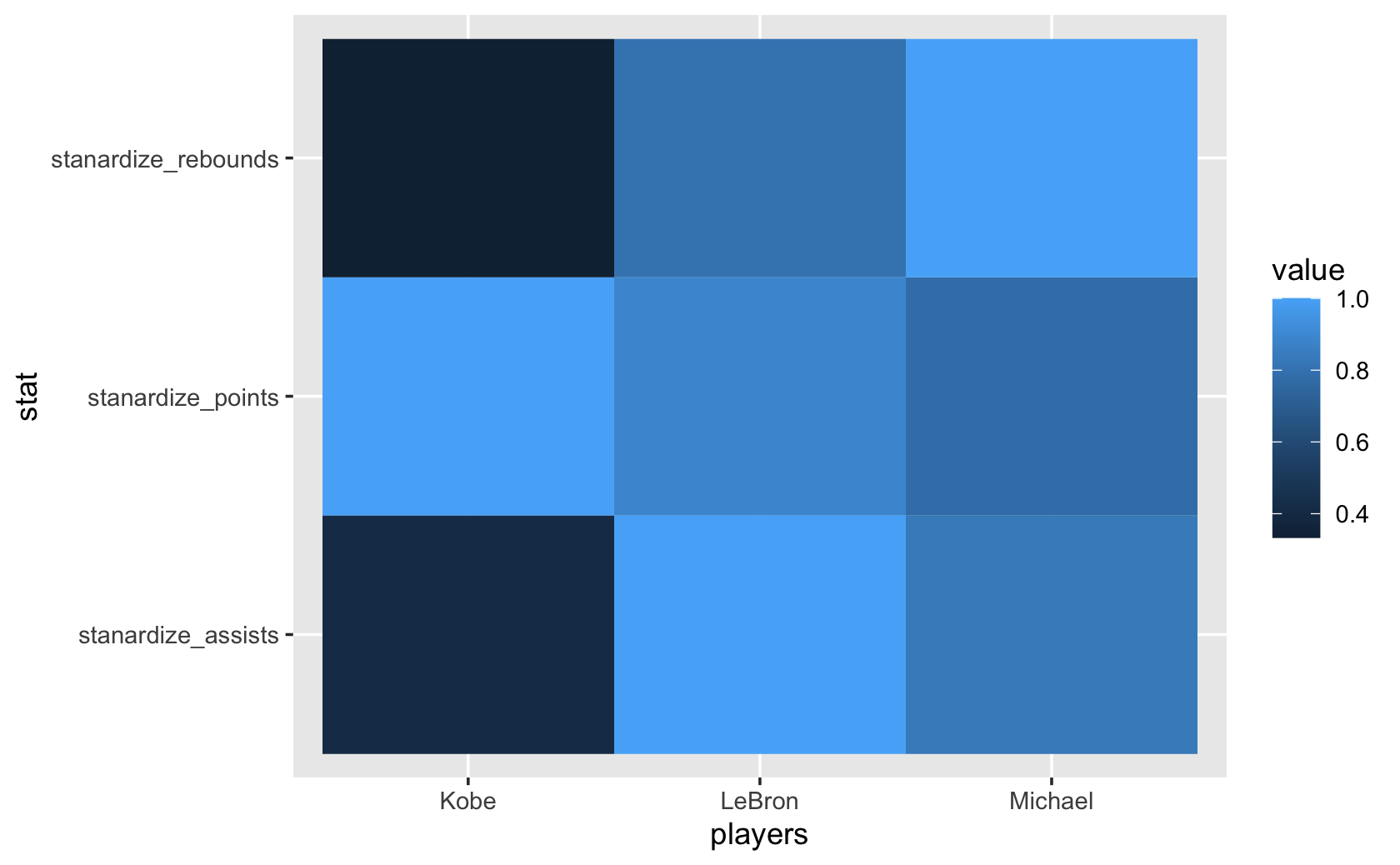# Part 10 Week 5

Data Visualization in R with ggplot2 > Week 2

## 10.1 Bar Plots Part 1, 2

``````library(tidyverse)

cel <-
url(
"https://www.dropbox.com/s/4ebgnkdhhxo5rac/cel_volden_wiseman%20_coursera.csv?raw=1"
)
)``````
``````#>
#> ── Column specification ─────────────────────────────────────────────
#> cols(
#>   .default = col_double(),
#>   thomas_name = col_character(),
#>   st_name = col_character()
#> )
#> ℹ Use `spec()` for the full column specifications.``````
``````####bar plot for dems variable in the 115th Congress. 0=Republican, 1=Democrat

cel %>%
filter(congress == 115) %>%
ggplot(aes(x = dem)) +
geom_bar()``````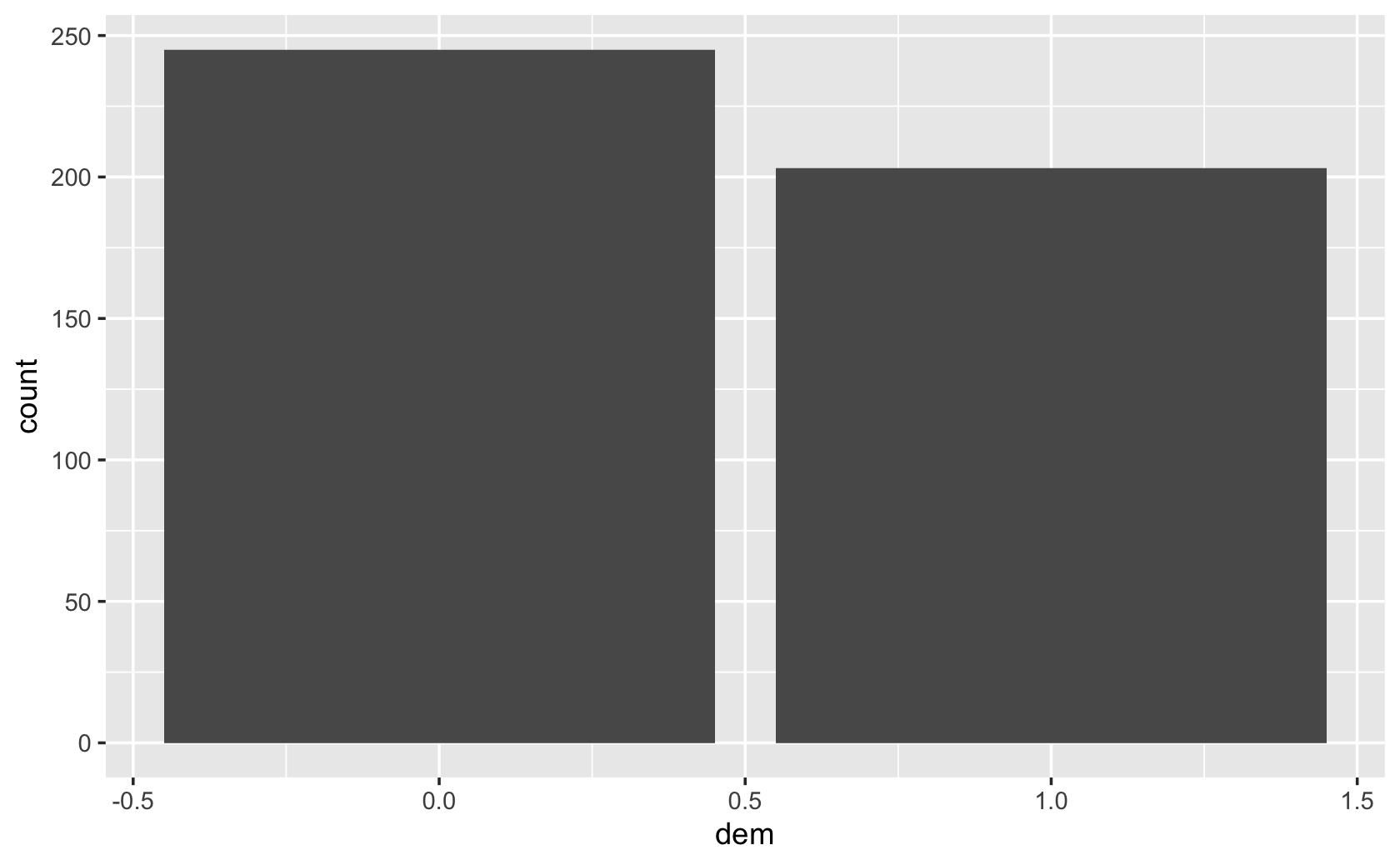``````###prove to yourself your bar plot is right by comparing with a frequency table:
table(filter(cel, congress == 115)\$dem)``````
``````#>
#>   0   1
#> 245 203``````
``````###use st_name instead, so how counts of how many members of Congress from each state:

cel %>% filter(congress == 115) %>% ggplot(aes(x = st_name)) + geom_bar()``````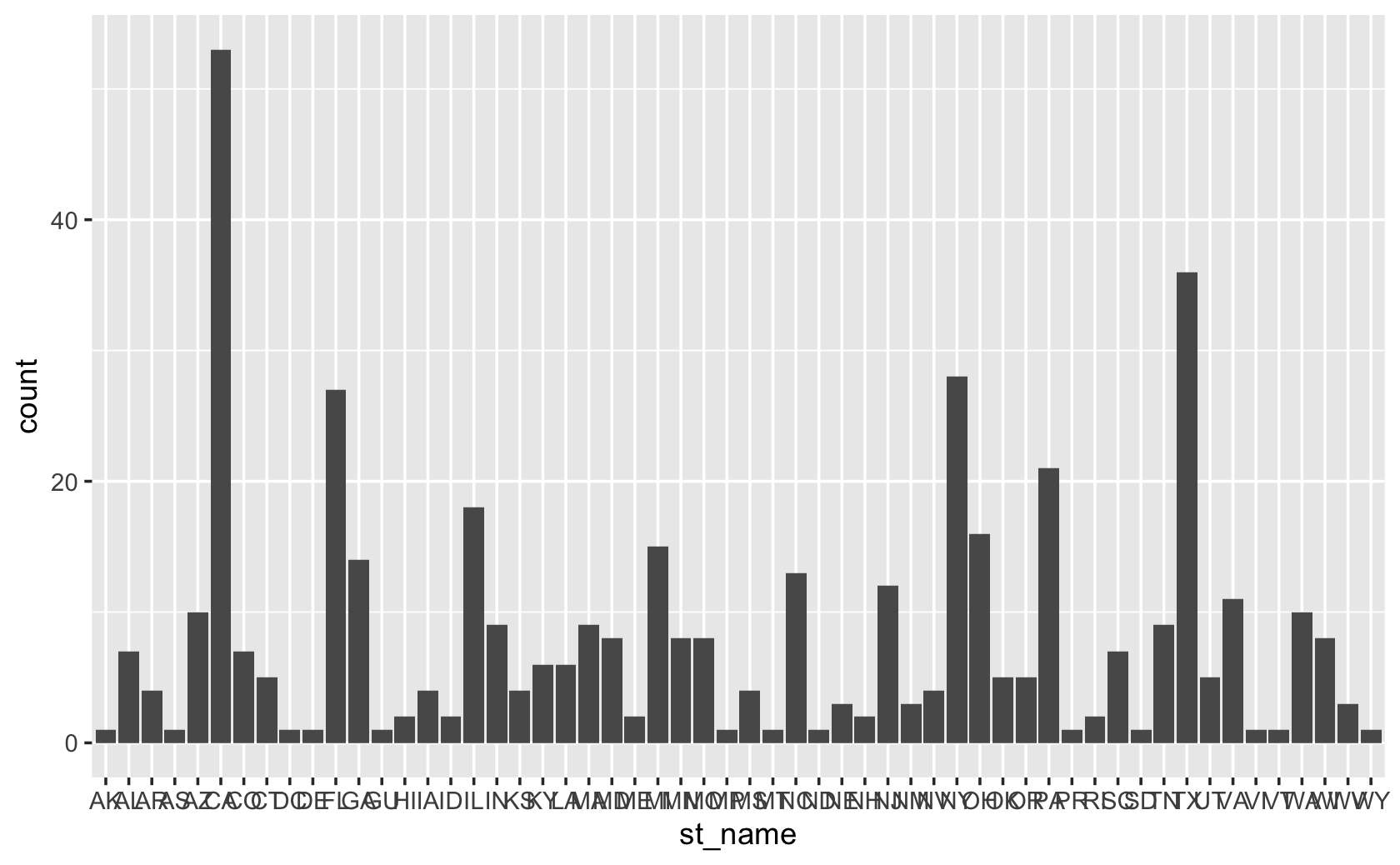``````###flip the figure by setting y aesthetic rather than the x

cel %>% filter(congress == 115) %>% ggplot(aes(y = st_name)) + geom_bar()``````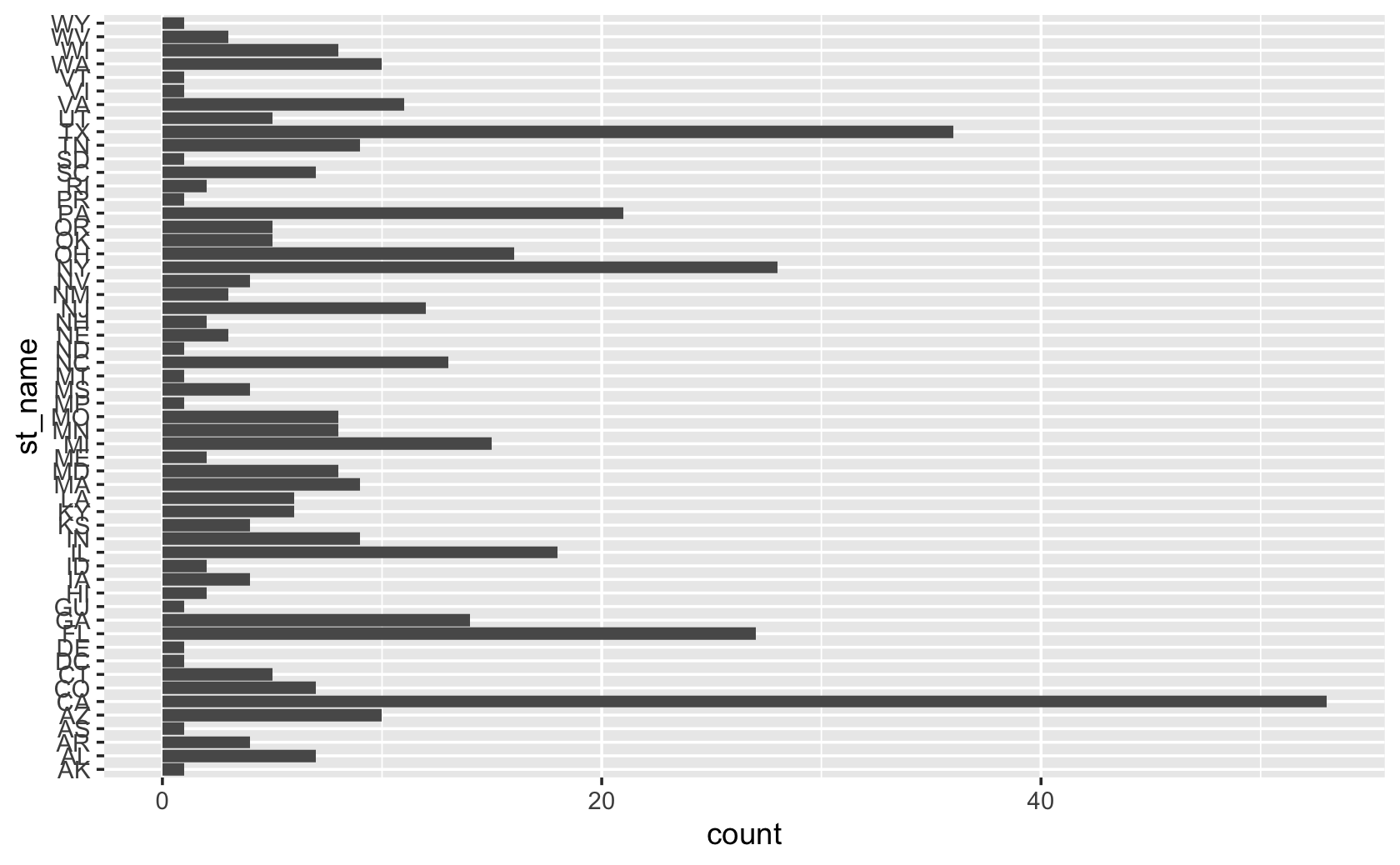``````###let's go back and recode the dem variable to be a categorical variable

party <- recode(cel\$dem, `1` = "Democrat", `0` = "Republican")

cel %>% filter(congress == 115) %>% ggplot(aes(x = party)) +
geom_bar()``````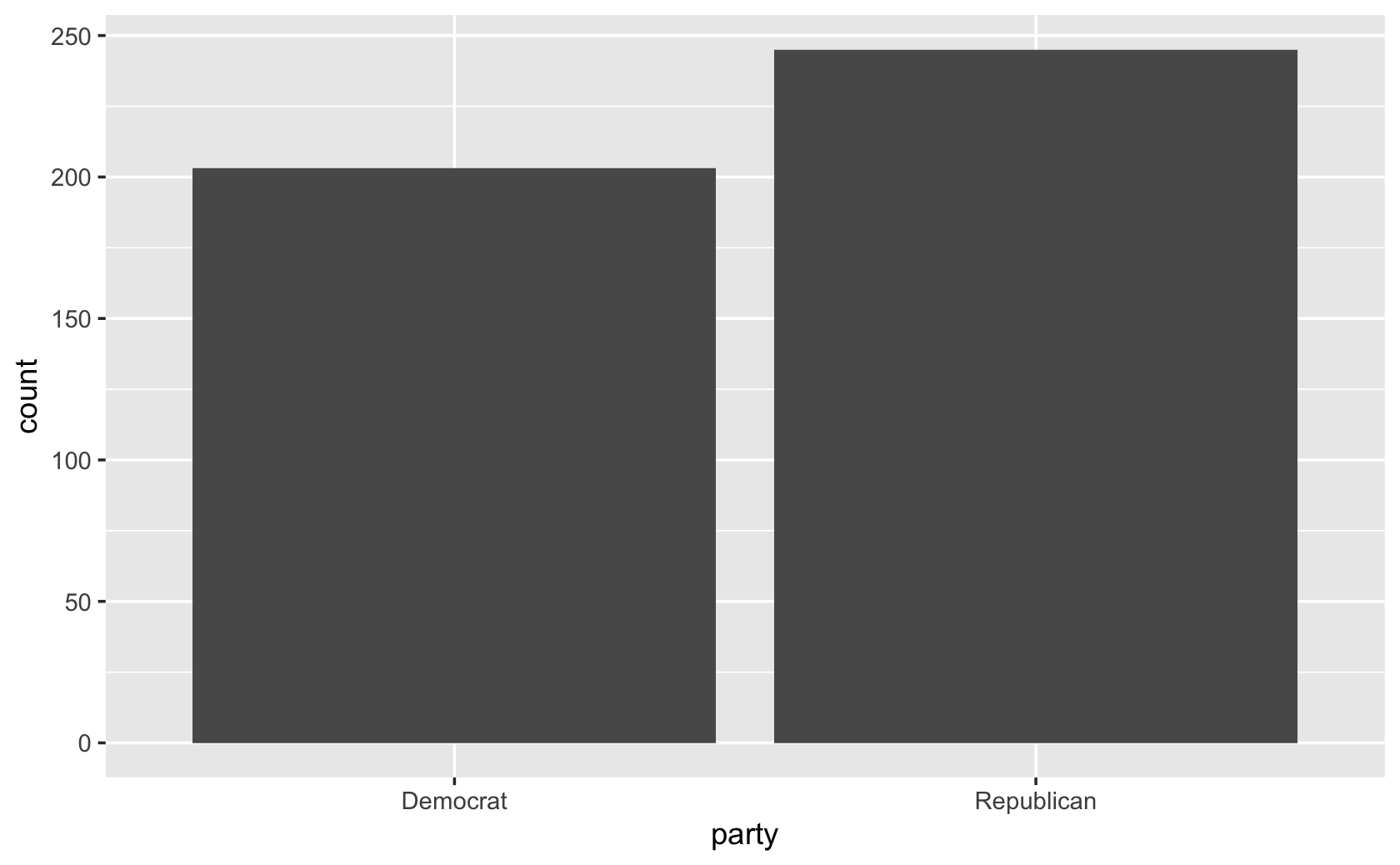``````####now add some visual touches

cel %>% filter(congress == 115) %>% ggplot(aes(x = party)) +
geom_bar() +
labs(x = "Party", y = "Number of Members")``````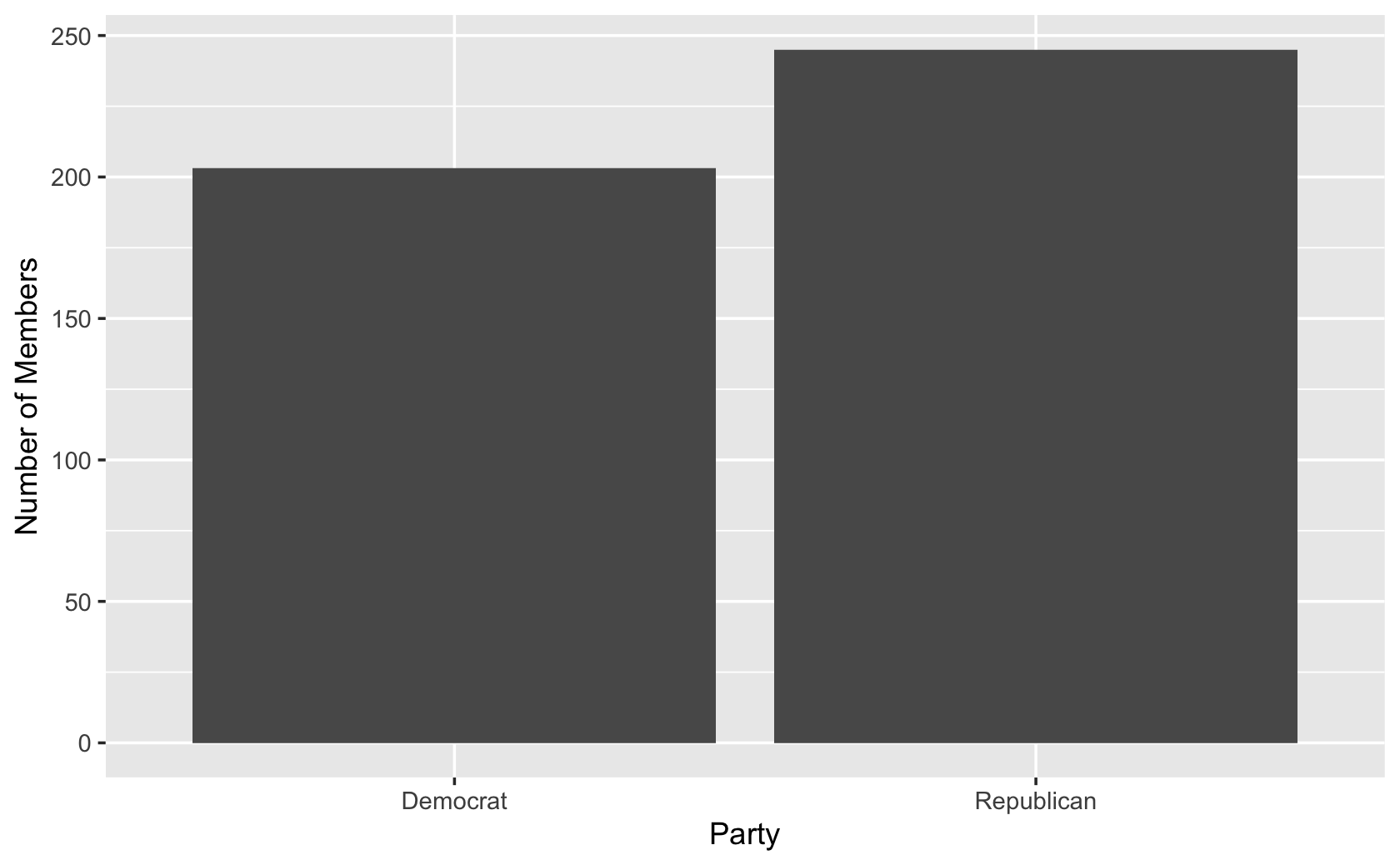``````###add colors for the two different bars
cel %>% filter(congress == 115) %>% ggplot(aes(x = party, fill = party)) +
geom_bar() +
labs(x = "Party", y = "Number of Members")``````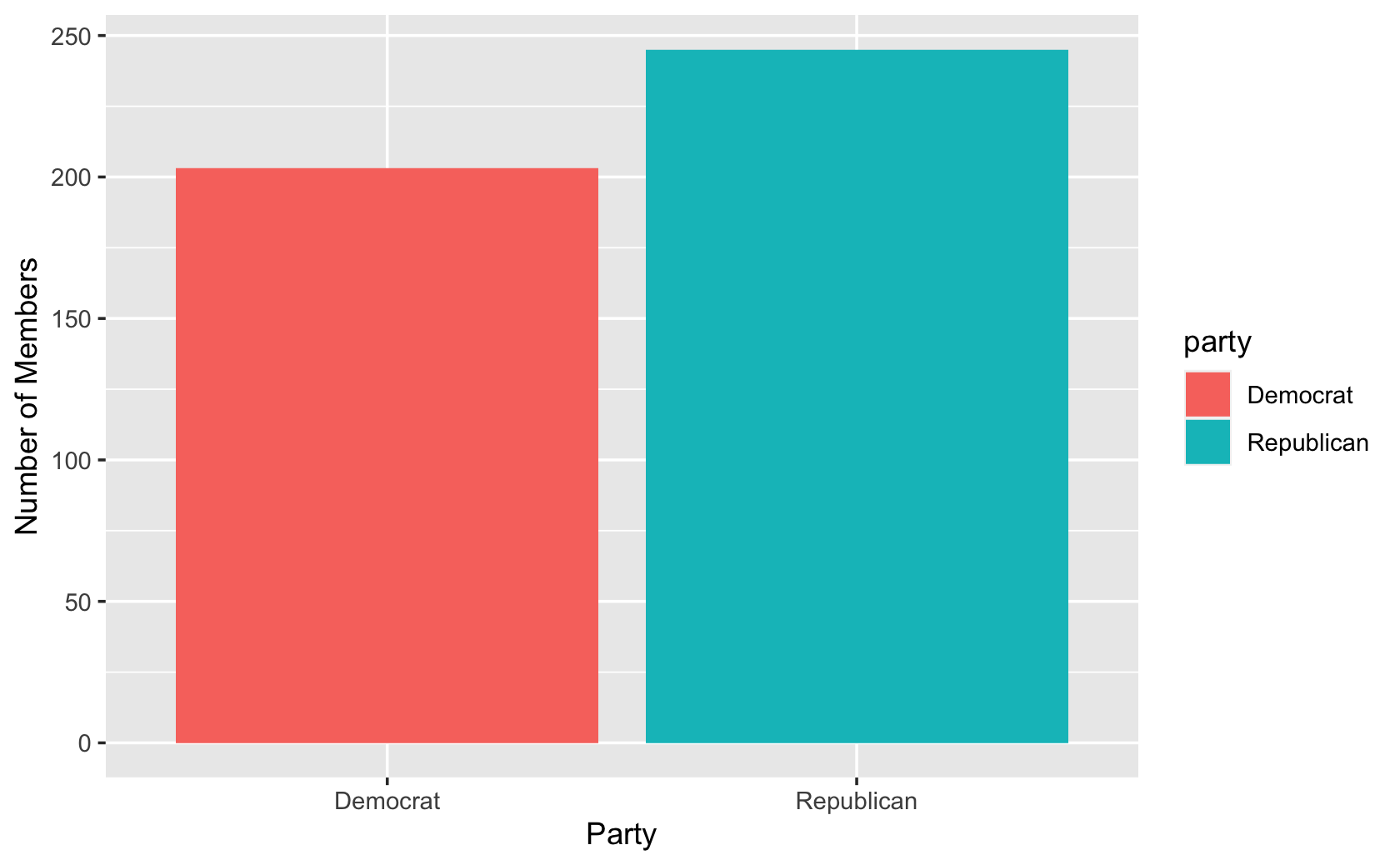``````###manually change the colors of the bars
cel %>% filter(congress == 115) %>% ggplot(aes(x = party, fill = party)) +
geom_bar() +
labs(x = "Party", y = "Number of Members") +
scale_fill_manual(values = c("blue", "red"))``````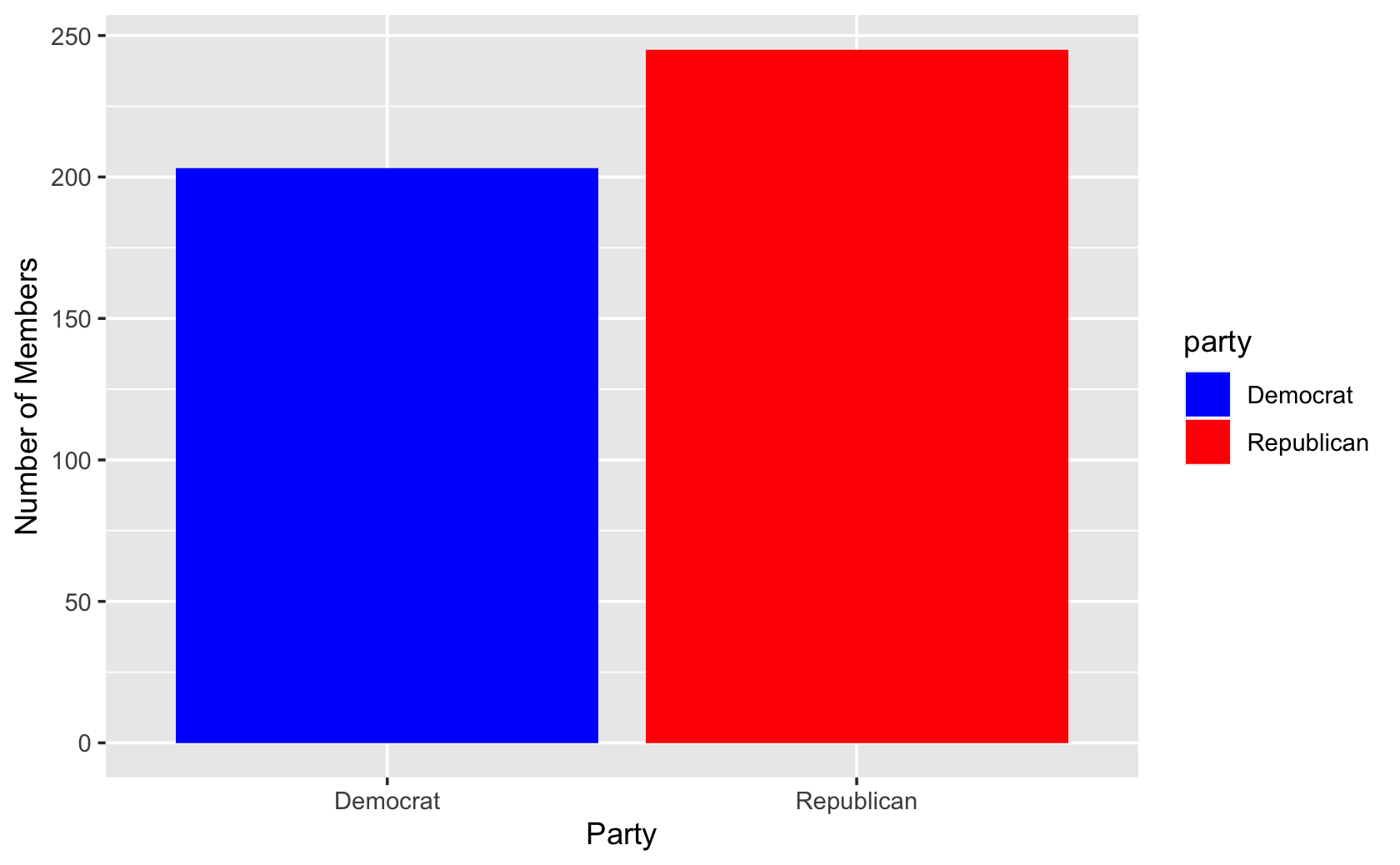``````###drop the legend with the "guides" command
cel %>% filter(congress == 115) %>% ggplot(aes(x = party, fill = party)) +
geom_bar() +
labs(x = "Party", y = "Number of Members") +
scale_fill_manual(values = c("blue", "red")) +
guides(fill = FALSE)``````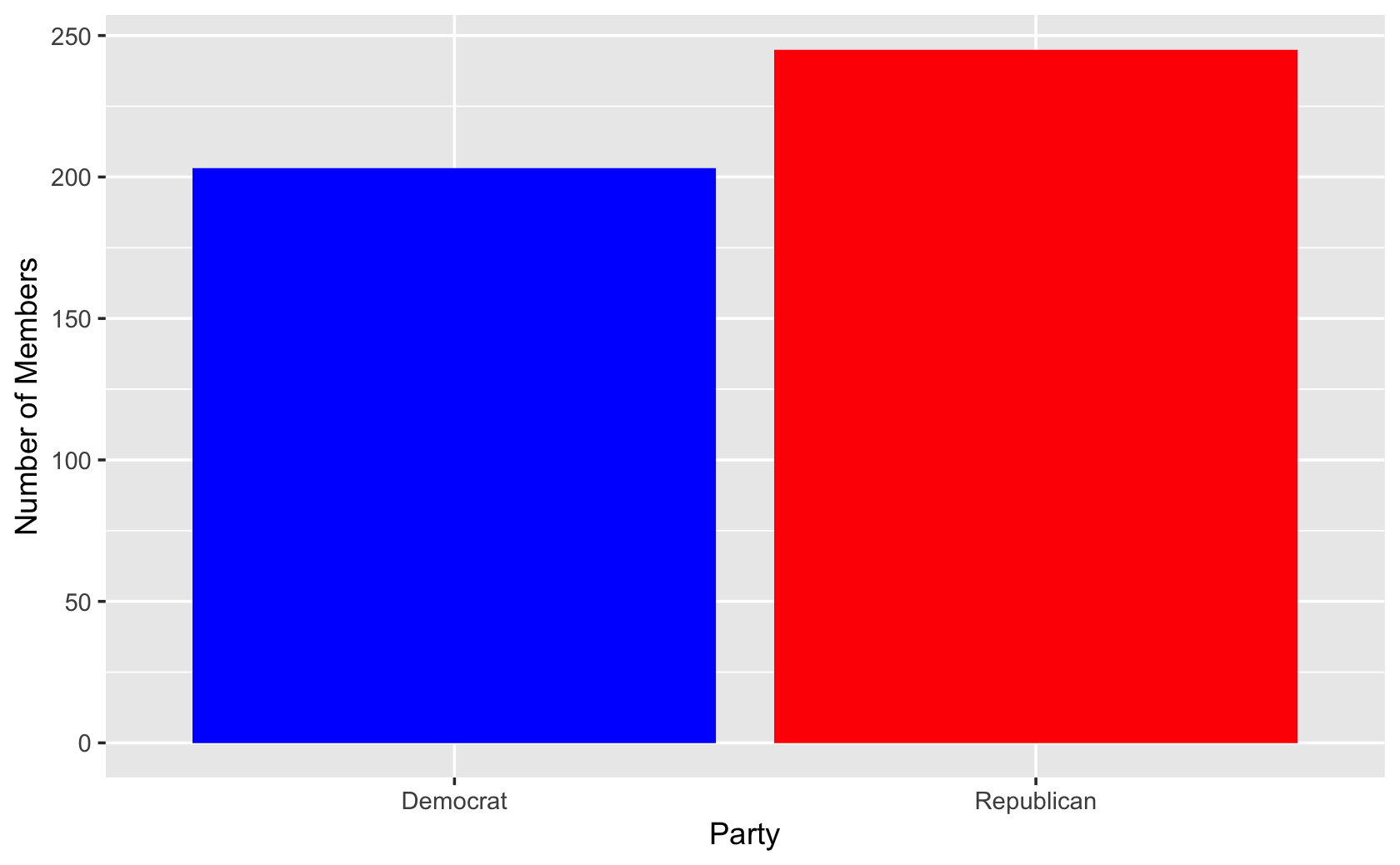``````#####Making more barplots and manipulating more data in R

####Making a barplot of proportions

#####a toy demonstration
#####a bowl of fruit
apple <- rep("apple", 6)
orange <- rep("orange", 3)
banana <- rep("banana", 1)

###put together the fruits in a dataframe
###creates a single columns with fruits
fruit_bowl <- tibble("fruits" = c(apple, orange, banana))

#####create a table that counts fruits in a second column
fruit_bowl_summary <- fruit_bowl %>%
group_by(fruits) %>%
summarize("count" = n())

fruit_bowl_summary``````
``````#> # A tibble: 3 x 2
#>   fruits count
#>   <chr>  <int>
#> 1 apple      6
#> 2 banana     1
#> 3 orange     3``````
``````####calculate proportions
fruit_bowl_summary\$proportion <-
fruit_bowl_summary\$count / sum(fruit_bowl_summary\$count)

fruit_bowl_summary``````
``````#> # A tibble: 3 x 3
#>   fruits count proportion
#>   <chr>  <int>      <dbl>
#> 1 apple      6        0.6
#> 2 banana     1        0.1
#> 3 orange     3        0.3``````
``````####add the geom_bar, using "stat" to tell command to plot the exact value for proportion
ggplot(fruit_bowl_summary, aes(x = fruits, y = proportion)) +
geom_bar(stat = "identity")``````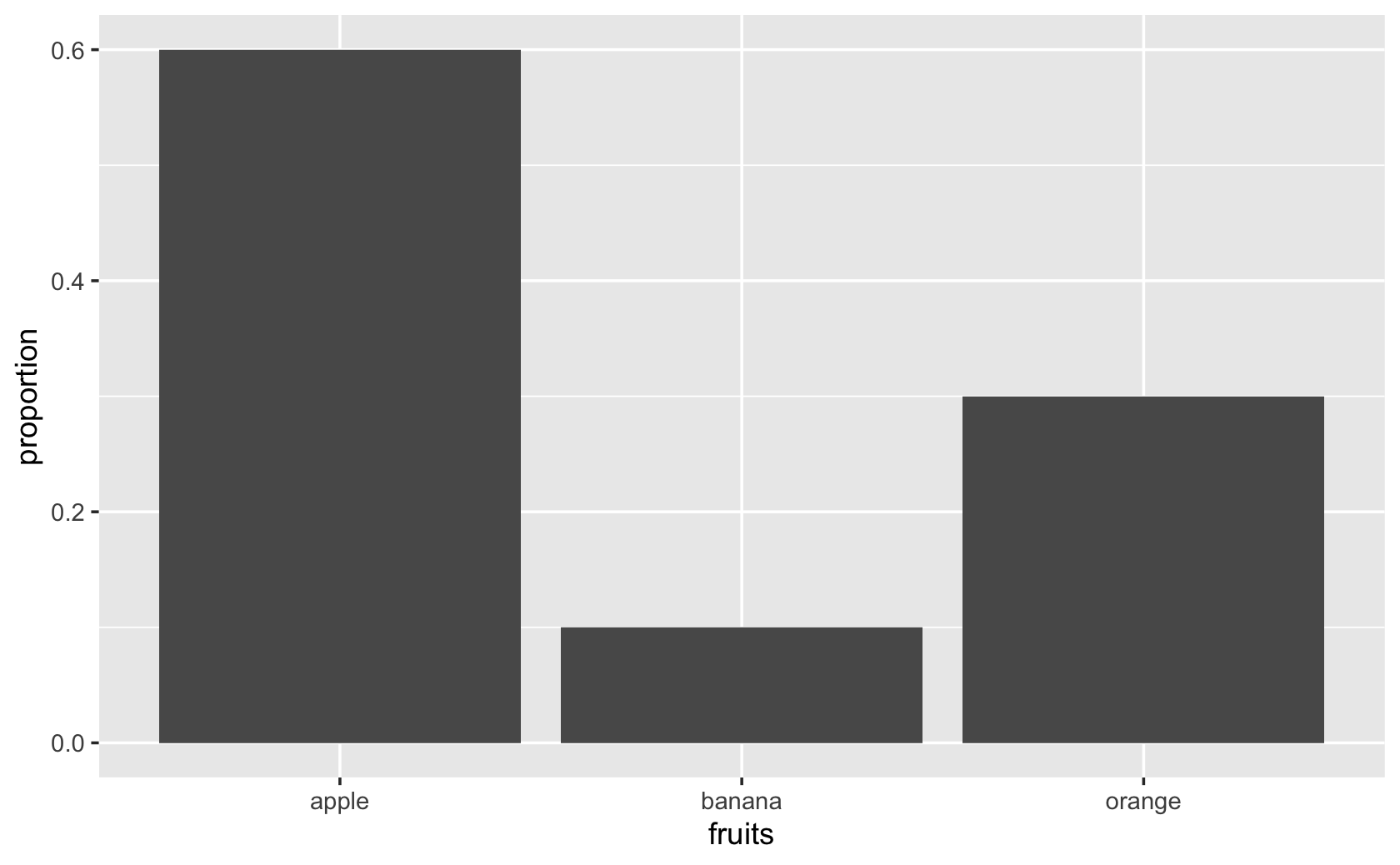``````ggplot(fruit_bowl_summary, aes(x = fruits, y = proportion, fill = fruits)) +
geom_bar(stat = "identity") +
scale_fill_manual(values = c("red", "yellow", "orange")) +
guides(fill = FALSE) +
labs(x = "Fruits", y = "Proportion of Fruits")``````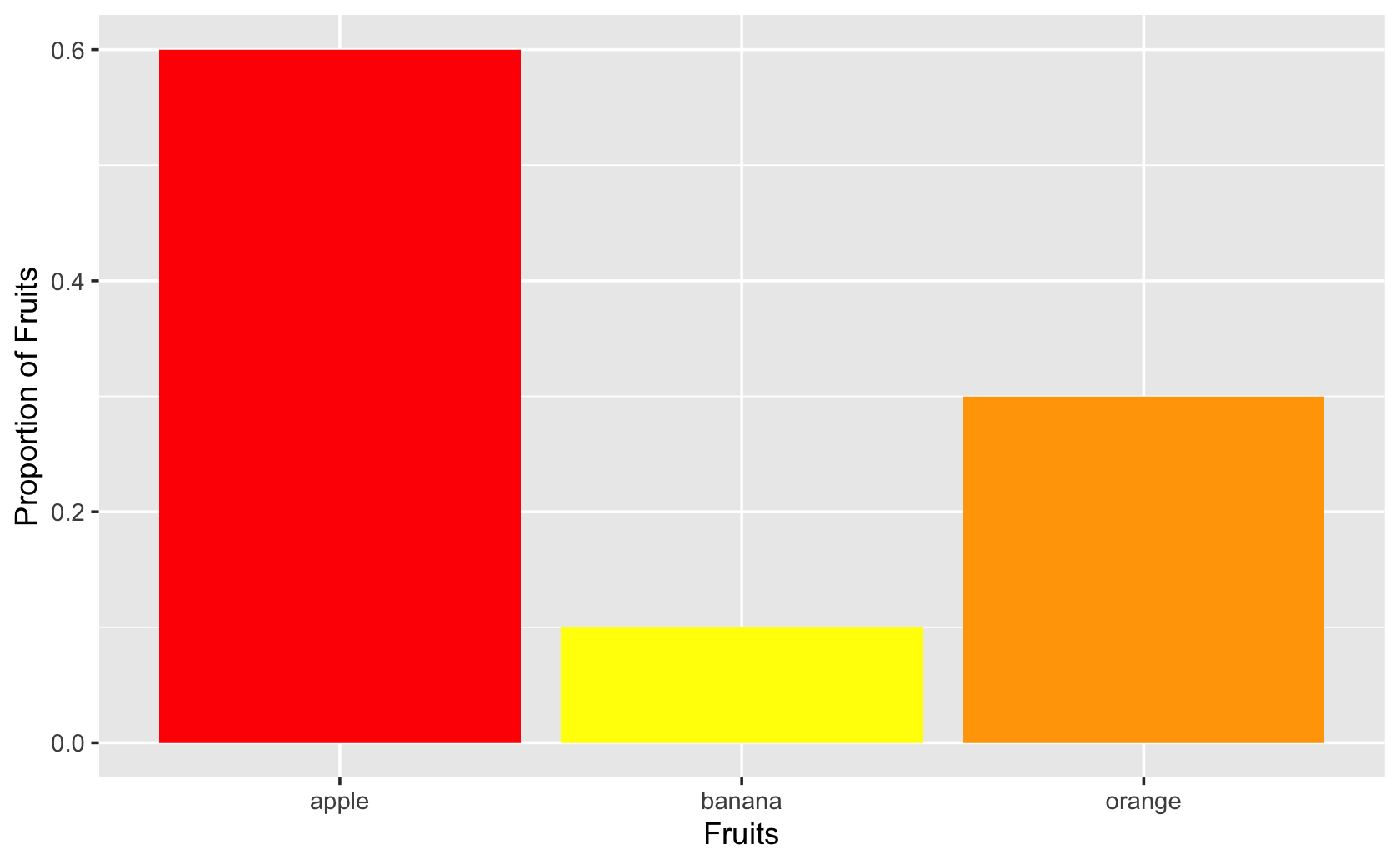``````####More practice with barplots!

#####
cces <-
url(
"https://www.dropbox.com/s/ahmt12y39unicd2/cces_sample_coursera.csv?raw=1"
)
)``````
``````#>
#> ── Column specification ─────────────────────────────────────────────
#> cols(
#>   .default = col_double()
#> )
#> ℹ Use `spec()` for the full column specifications.``````
``````####create counts of Ds, Rs, and Is by region

dem_rep <-
recode(
cces\$pid7,
`1` = "Democrat",
`2` = "Democrat",
`3` = "Democrat",
`4` = "Independent",
`5` = "Republican",
`6` = "Republican",
`7` = "Republican"
)

table(dem_rep)``````
``````#> dem_rep
#>    Democrat Independent  Republican
#>         516         119         365``````
``````cces <- add_column(cces, dem_rep)

###stacked bars
ggplot(cces, aes(x = region, fill = dem_rep)) +
geom_bar()``````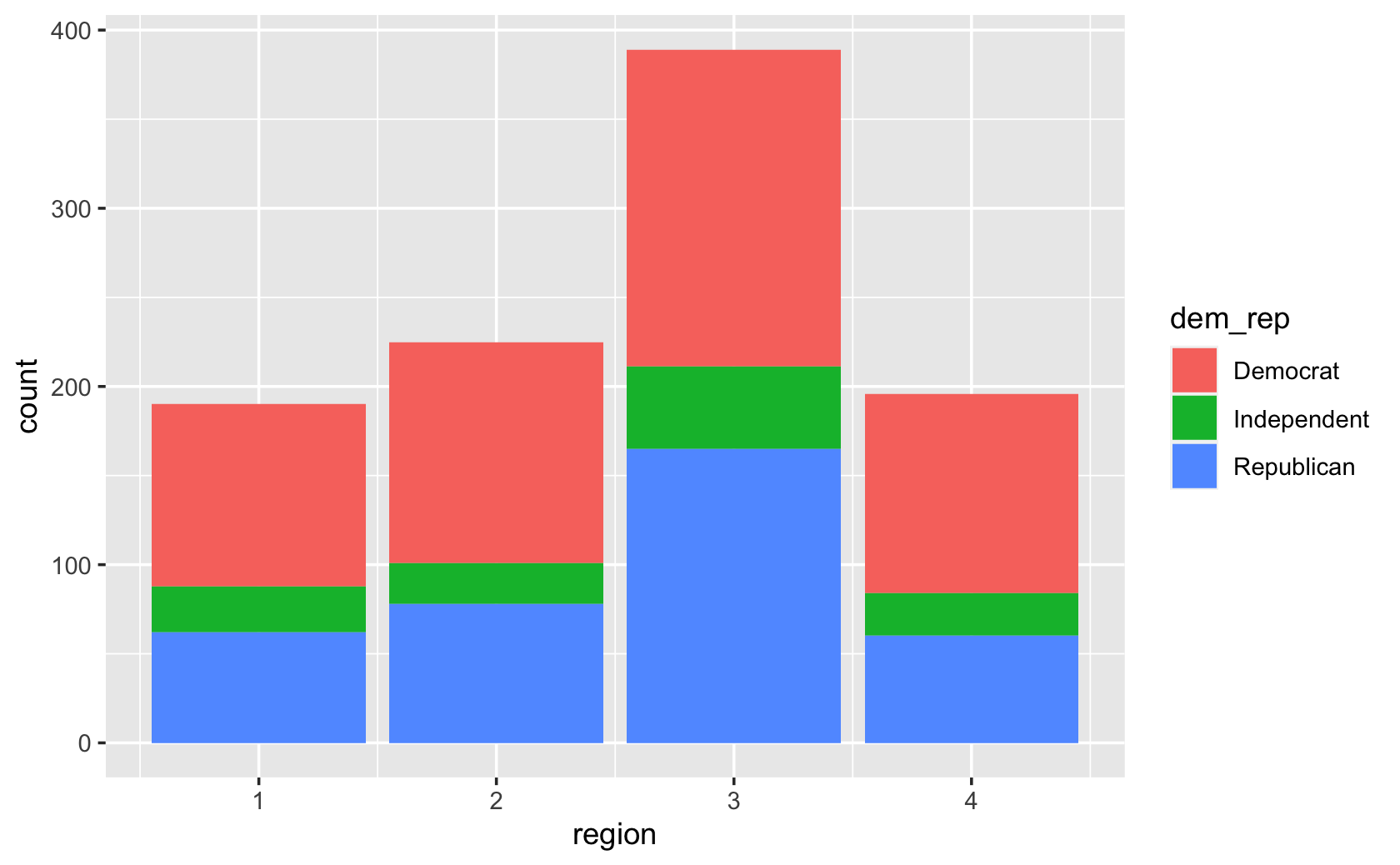``````###grouped bars
ggplot(cces, aes(x = region, fill = dem_rep)) +
geom_bar(position = "dodge")``````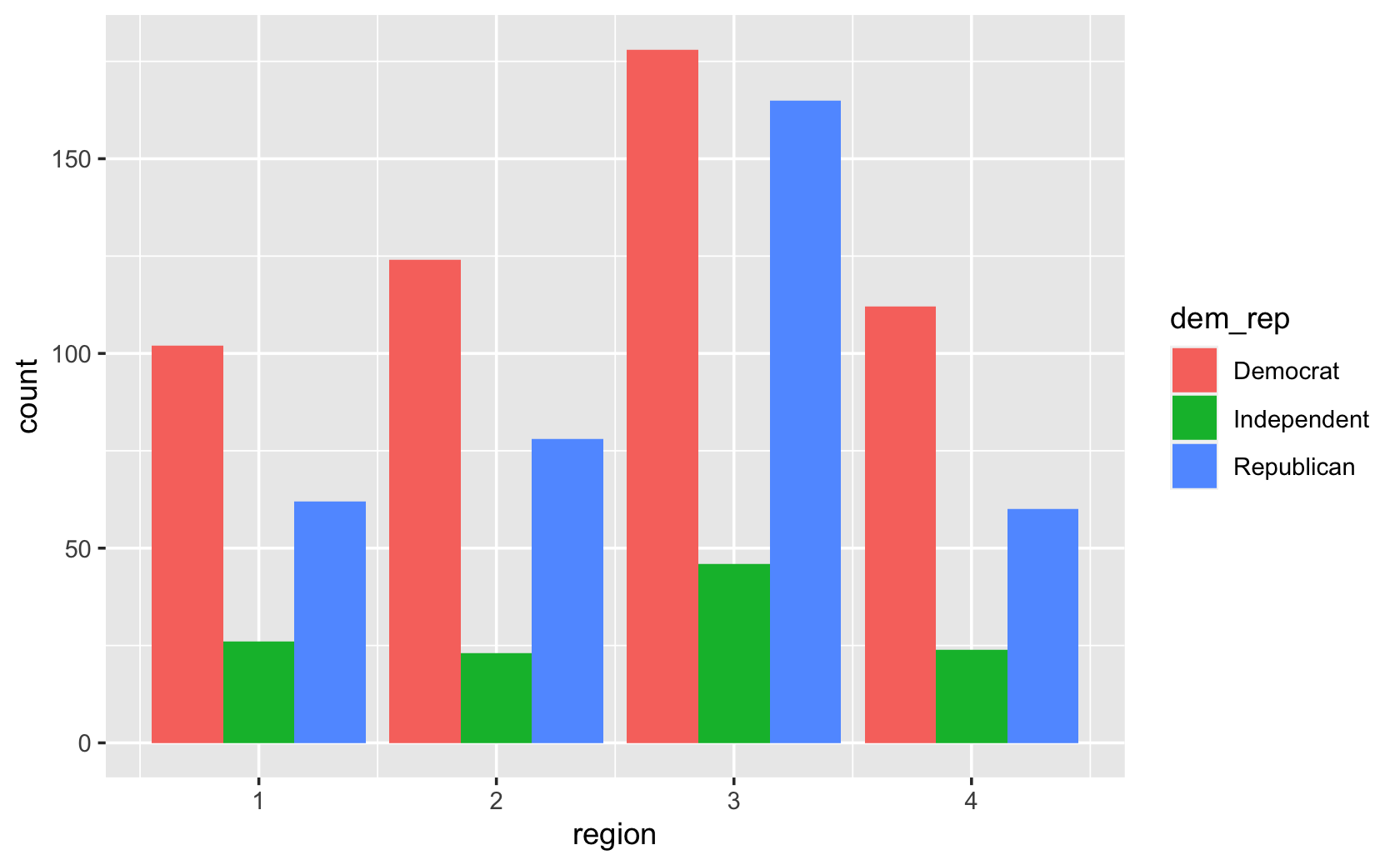``````##visual touches like relabeling the axes
ggplot(cces, aes(x = region, fill = dem_rep)) +
geom_bar(position = "dodge") +
labs(x = "Region", y = "Count")``````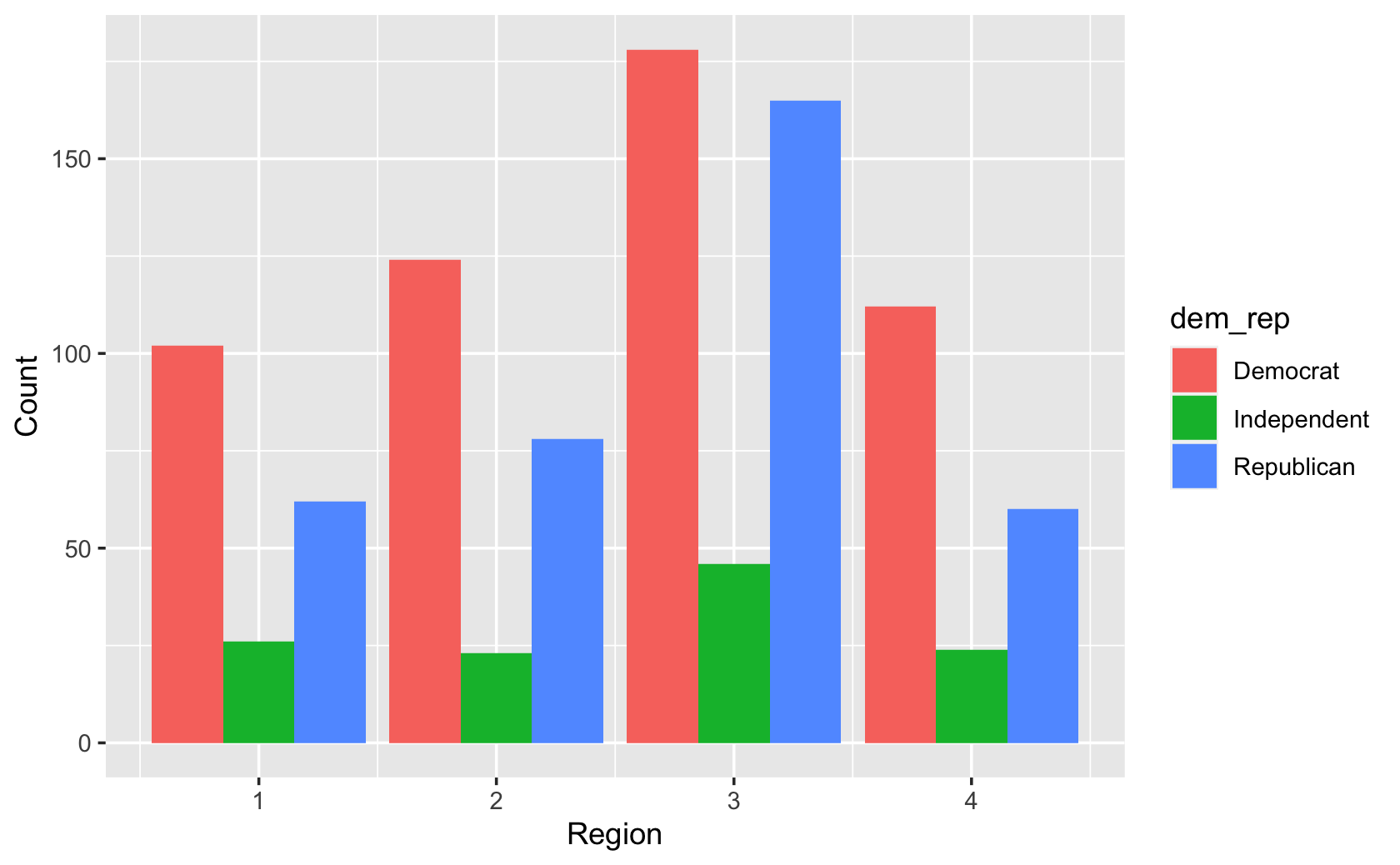## 10.2 Line Plots Part 1

``````library(tidyverse)

####create a sequence of years

years <- seq(from = 2001, to = 2020, by = 1)

####create "fake" data for price (note, your values will be different)

price <- rnorm(20, mean = 15, sd = 5)

####put years and price together

fig_data <- tibble("year" = years, "stock_price" = price)

ggplot(fig_data, (aes(x = years, y = price))) +
geom_line()``````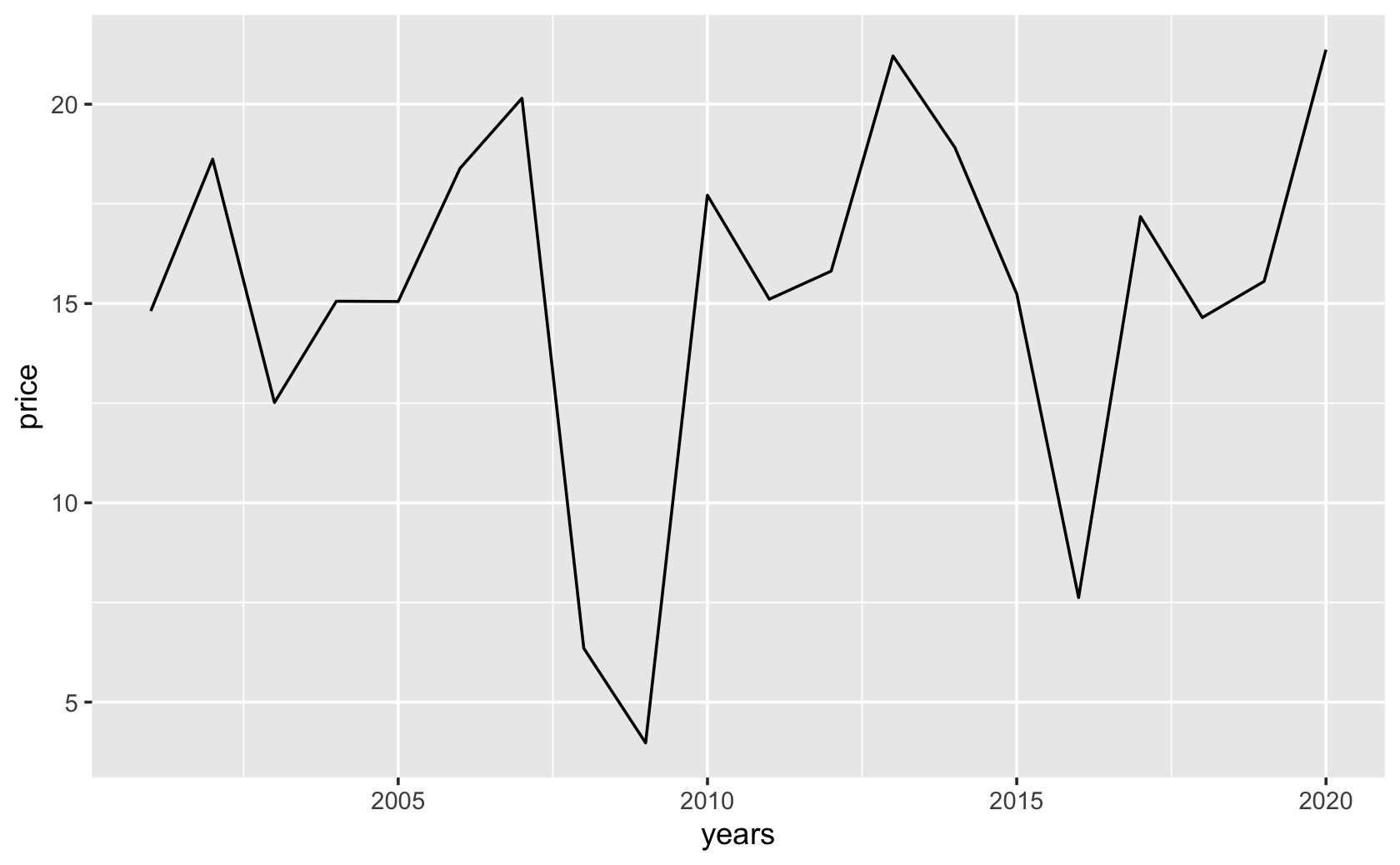``````####make data for the first of two stocks

fig_data\$stock_id = rep("Stock_1", 20)

stock_1_time_series <- fig_data

#####create data for the second company
########same approach as with the last company
stock_id <- rep("Stock_2", 20)

years <- seq(from = 2001, to = 2020, by = 1)

price <- rnorm(20, mean = 10, sd = 3)

stock_2_time_series <-
tibble("stock_id" = stock_id,
"year" = years,
"stock_price" = price)

####combine with bind_rows()

all_stocks_time_series <-
bind_rows(stock_1_time_series, stock_2_time_series)

# View(all_stocks_time_series)

####make the plot, setting group to stock_id

ggplot(all_stocks_time_series, (aes(
x = year, y = stock_price, group = stock_id
))) +
geom_line()``````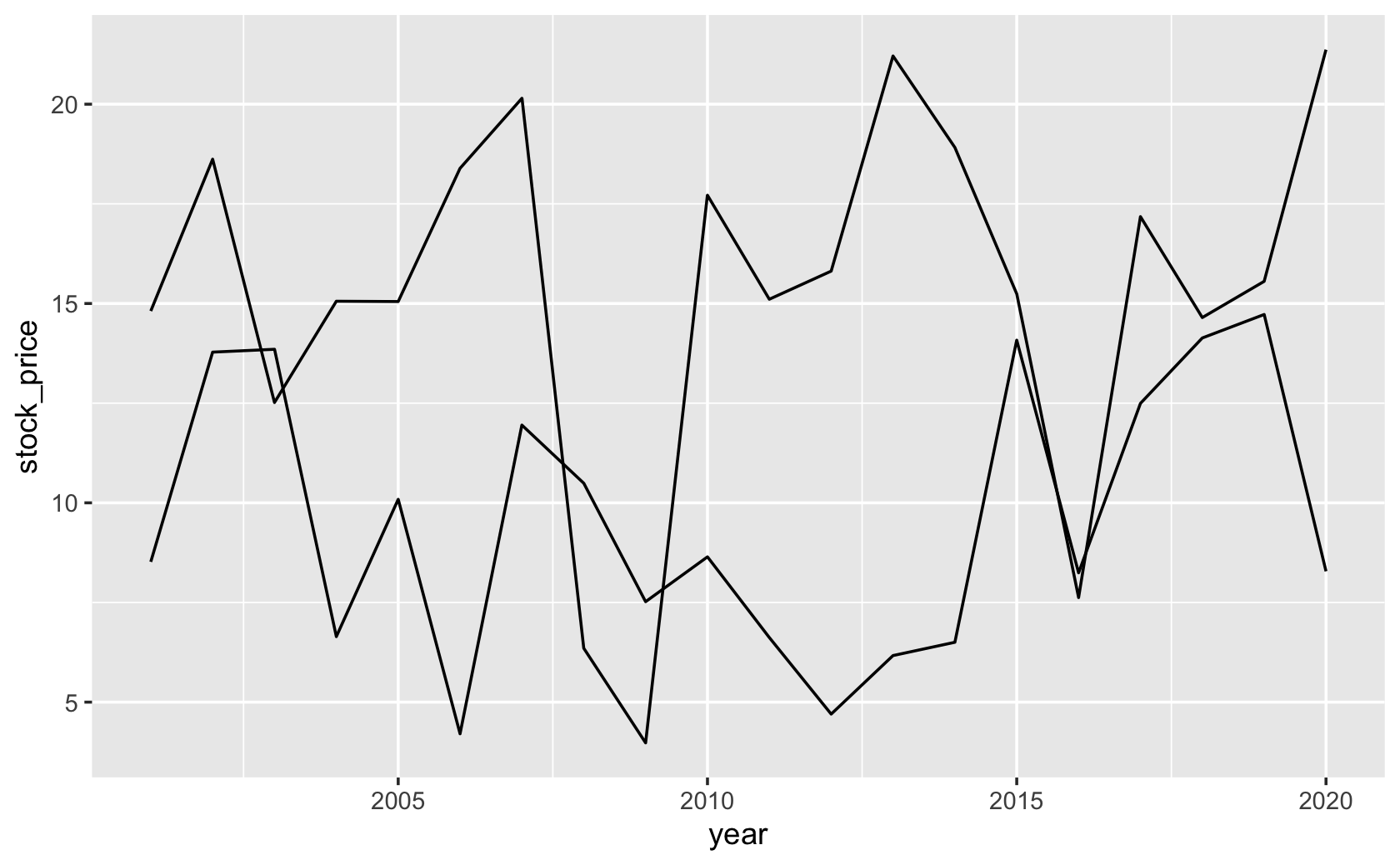``````####modify group, linetype, color, and add facet_wrap()

ggplot(all_stocks_time_series, (
aes(
x = year,
y = stock_price,
group = stock_id,
linetype = stock_id,
color = stock_id
)
)) +
geom_line() +
facet_wrap( ~ stock_id)``````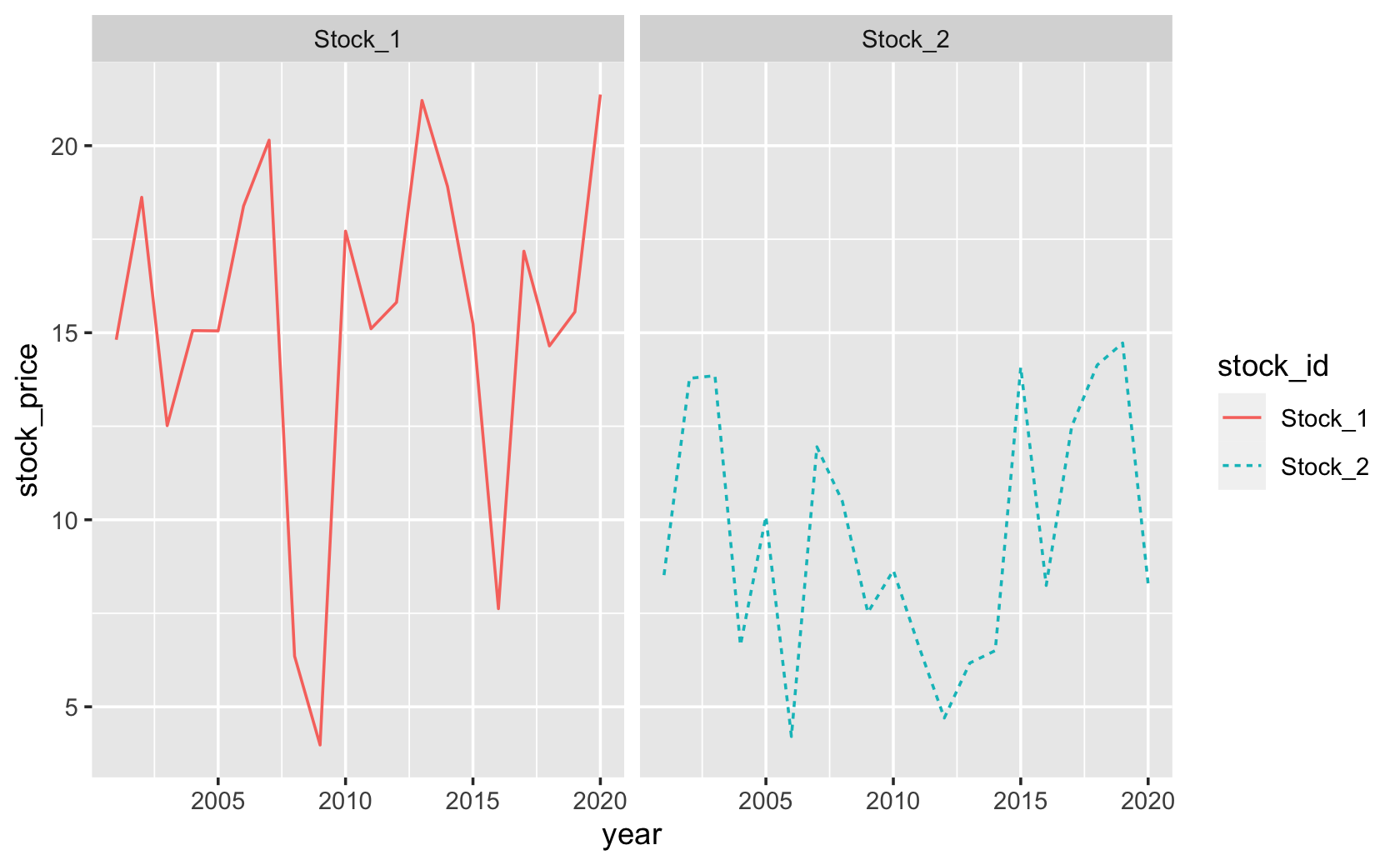``````#####Practice with another data set

cel <-
url(
"https://www.dropbox.com/s/4ebgnkdhhxo5rac/cel_volden_wiseman%20_coursera.csv?raw=1"
)
)``````
``````#>
#> ── Column specification ─────────────────────────────────────────────
#> cols(
#>   .default = col_double(),
#>   thomas_name = col_character(),
#>   st_name = col_character()
#> )
#> ℹ Use `spec()` for the full column specifications.``````
``````cel\$Party <- recode(cel\$dem, `1` = "Democrat", `0` = "Republican")

fig_data <- cel %>%
group_by(Party, year) %>%
summarize("Ideology" = mean(dwnom1, na.rm = T))``````
``#> `summarise()` has grouped output by 'Party'. You can override using the `.groups` argument.``
``````# View(fig_data)

ggplot(fig_data, (aes(
x = year,
y = Ideology,
group = Party,
color = Party
))) +
geom_line() +
scale_color_manual(values = c("blue", "red"))``````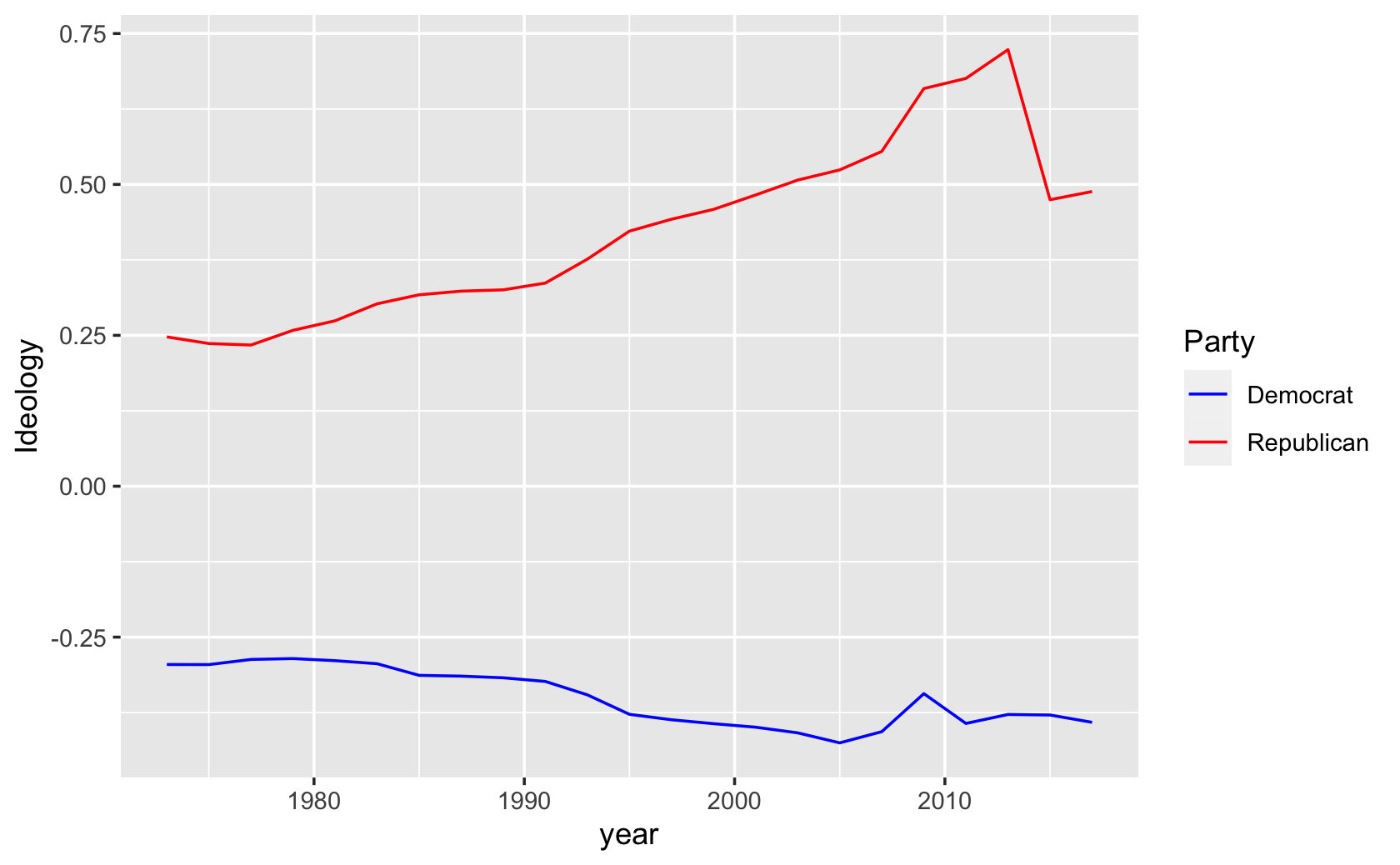## 10.3 Learning New Figures Part 1

``````# Library
library(tidyverse)

# Dummy data
x <- LETTERS[1:20]
y <- paste0("var", seq(1, 20))

# ? expand.grid
dat <- expand.grid(X = x, Y = y)

# ? runif
dat\$Z <- runif(400, 0, 5)

# Heatmap
ggplot(dat, aes(x = X, y = Y, fill = Z)) +
geom_tile()``````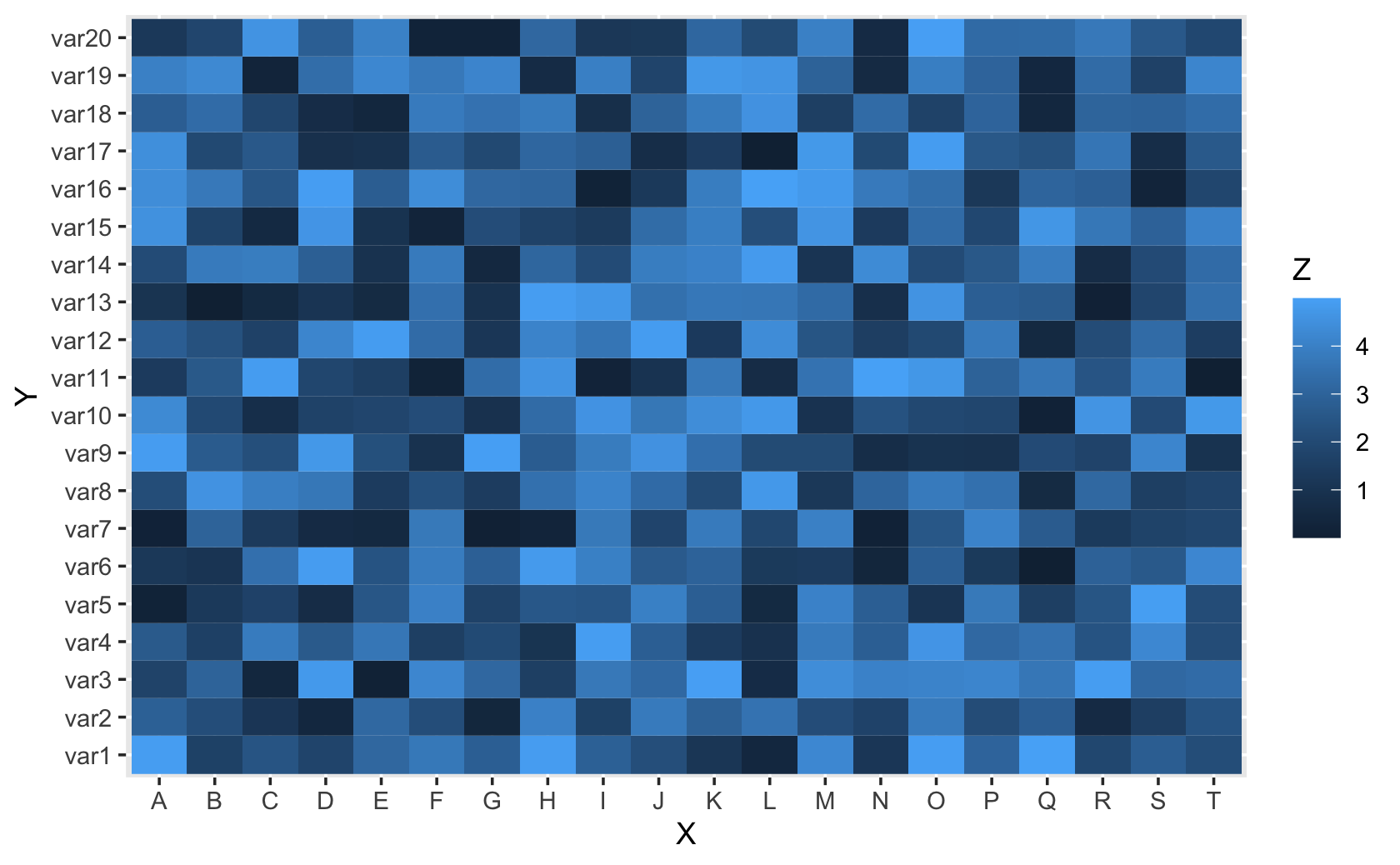``````#####practice again using a more substantive example

players <- c("Michael", "LeBron", "Kobe")
points <- c(35, 40, 45)
assists <- c(10, 12, 5)
rebounds <- c(15, 12, 5)

basketball <- tibble(players, points, assists, rebounds)

#####standardize the values

select(
"players",
"stanardize_points",
"stanardize_assists",
"stanardize_rebounds"
)

``````#> # A tibble: 3 x 4
#>   players stanardize_points stanardize_assists stanardize_rebounds
#>   <chr>               <dbl>              <dbl>               <dbl>
#> 1 Michael             0.778              0.833               1
#> 2 LeBron              0.889              1                   0.8
#> 3 Kobe                1                  0.417               0.333``````
``````long_basketball_scaled <-
pivot_longer(
c(
"stanardize_points",
"stanardize_assists",
"stanardize_rebounds"
),
names_to = "stat",
values_to = "value"
)

``````#> # A tibble: 9 x 3
#>   players stat                value
#>   <chr>   <chr>               <dbl>
#> 1 Michael stanardize_points   0.778
#> 2 Michael stanardize_assists  0.833
#> 3 Michael stanardize_rebounds 1
#> 4 LeBron  stanardize_points   0.889
#> 5 LeBron  stanardize_assists  1
#> 6 LeBron  stanardize_rebounds 0.8
#> # … with 3 more rows``````
``````ggplot(long_basketball_scaled, aes(x = players, y = stat, fill = value)) +
geom_tile()``````